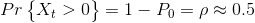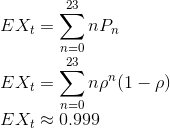Math Modeling : Markov Chains & Processes

Example Questions

Example Question #1 : Stochastic Models

A computer company has one service repair man and has space for 29 computers in the shop at one time. Last year the shop repaired 67 computers with an average repair time of 2 days per computer. Formulate a Markov process model for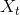which represents the number of computers in the shop for repair at timemonths and calculate the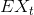.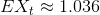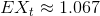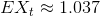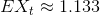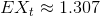Explanation:

Using Markov Process for this question assume that the space is finite.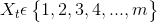The stochastic process which determines the future of the process from a probabilistic standpoint is defined as follows.

Let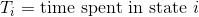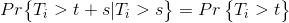Recall that the exponential distribution, and thus the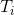could have the density function,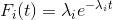Now formulate the Markov Process specifically for this problem.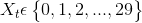The transitions of computers in and out of the shop are,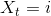to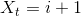or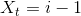Now calculate the rates up and down.

To calculate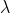take the total number of computers fixed last year and divide it by the total months in the year. To calculate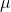assume there are 22 working days in a month and it takes on average, two days to fix a computer.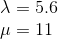Its key to know that at zero we cannot move down a state and like wise when at 29, we cannot move up a state.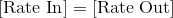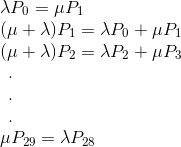Solving with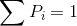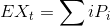Continuing in this fashion results in the following.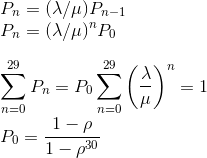Now, writing the sum of a finite geometric series is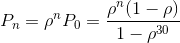Now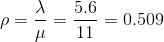therefore,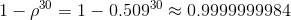Next,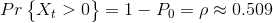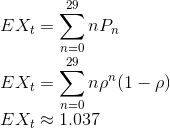Example Question #2 : Stochastic Models

A computer company has one service repair man and has space for 32 computers in the shop at one time. Last year the shop repaired 71 computers with an average repair time of 3 days per computer. Formulate a Markov process model forwhich represents the number of computers in the shop for repair at timemonths and calculate the.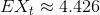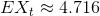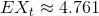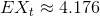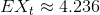Explanation:

Using Markov Process for this question assume that the space is finite.The stochastic process which determines the future of the process from a probabilistic standpoint is defined as follows.

LetRecall that the exponential distribution, and thus thecould have the density function,Now formulate the Markov Process specifically for this problem.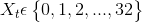The transitions of computers in and out of the shop are,toorNow calculate the rates up and down.

To calculatetake the total number of computers fixed last year and divide it by the total months in the year. To calculateassume there are 22 working days in a month and it takes on average, two days to fix a computer.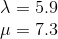Its key to know that at zero we cannot move down a state and like wise when at 29, we cannot move up a state.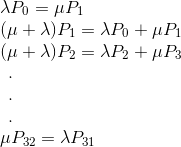Solving withContinuing in this fashion results in the following.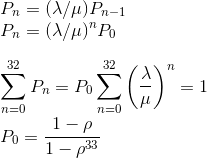Now, writing the sum of a finite geometric series isNow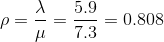therefore,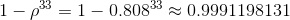Next,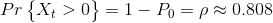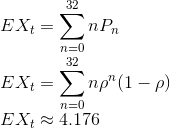Example Question #3 : Stochastic Models

A computer company has one service repair man and has space for 23 computers in the shop at one time. Last year the shop repaired 51 computers with an average repair time of 5 days per computer. Formulate a Markov process model forwhich represents the number of computers in the shop for repair at timemonths and calculate the.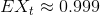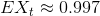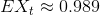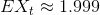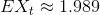Explanation:

Using Markov Process for this question assume that the space is finite.The stochastic process which determines the future of the process from a probabilistic standpoint is defined as follows.

LetRecall that the exponential distribution, and thus thecould have the density function,Now formulate the Markov Process specifically for this problem.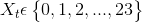The transitions of computers in and out of the shop are,toorNow calculate the rates up and down.

To calculatetake the total number of computers fixed last year and divide it by the total months in the year. To calculateassume there are 22 working days in a month and it takes on average, two days to fix a computer.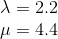Its key to know that at zero we cannot move down a state and like wise when at 29, we cannot move up a state.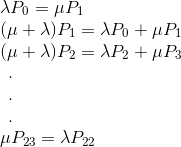Solving withContinuing in this fashion results in the following.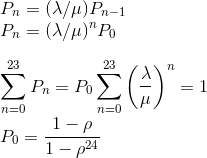Now, writing the sum of a finite geometric series isNow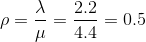therefore,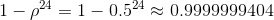Next,